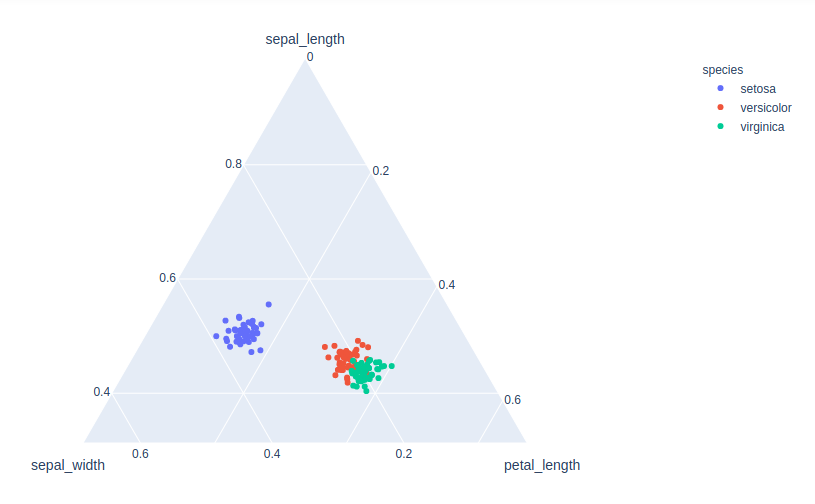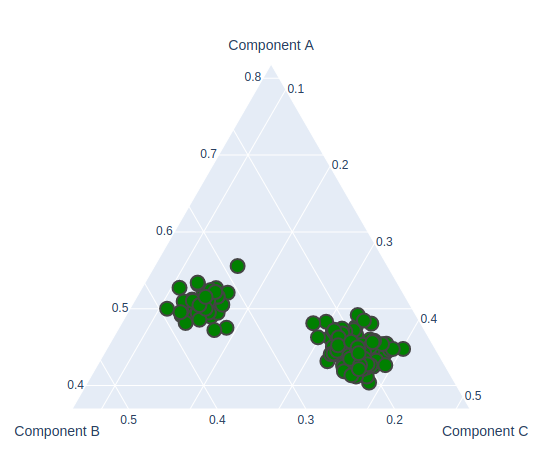# Ternary Plots in Plotly

A Plotly is a Python library that is used to design graphs, especially interactive graphs. It can plot various graphs and charts like histogram, barplot, boxplot, spreadplot, and many more. It is mainly used in data analysis as well as financial analysis. plotly is an interactive visualization library.

## Ternary Plots in Plotly

A ternary plot is also known as a ternary graph, triangle plot, simplex plot which is a barycentric plot on three variables which sum to a constant. It graphically depicts the ratios of the three variables as positions in an equilateral triangle. It can be created using two-class i.e. express class and graph_objects class.

### Using express class

In plotly, express is an easy-to-use,high-level interface that helps to operates a variety of types of data and produce simple style figures. It provides scatter_ternary() method to create ternary plots.

Syntax: scatter_ternary( a=None, b=None, c=None, color=None,labels={},width=None, height=None)

Parameters:

a: Values from this column or array_like are used to position marks along the a axis in ternary coordinates.

b: Values from this column or array_like are used to position marks along the b axis in ternary coordinates.

c: Values from this column or array_like are used to position marks along the c axis in ternary coordinates.

color:  Either a name of a column in data_frame, or a pandas Series or array_like object. Values from this column or array_like are used to assign color to marks.

width: The figure width in pixels.

height: The figure height in pixels.

Example:

## Python3

 `import` `plotly.express as px ` ` `  ` `  `df ``=` `px.data.iris() ` `fig ``=` `px.scatter_ternary(df, a``=``"sepal_length"``, ` `                         ``b``=``"sepal_width"``, c``=``"petal_length"``, ` `                         ``color``=``"species"``, size_max``=``20``) ` `fig.show() `

Output:### Using Graph_Objects class

Let’s see one example of a ternary scatter plot with plotly graph objects to make it clear. It can be created using the Scatterternary() method.

Example:

## Python3

 `import` `plotly.express as px ` `import` `plotly.graph_objects as go ` ` `  ` `  `df ``=` `px.data.iris() ` `fig ``=` `go.Figure(go.Scatterternary({ ` `    ``'mode'``: ``'markers'``, ` `    ``'a'``: df[``'sepal_length'``], ` `    ``'b'``: df[``'sepal_width'``], ` `    ``'c'``: df[``'petal_length'``], ` `    ``'marker'``: { ` `        ``'color'``: ``'green'``, ` `        ``'size'``: ``14``, ` `        ``'line'``: {``'width'``: ``2``} ` `    ``} ` `})) ` ` `  `fig.show() `

Output:Attention geek! Strengthen your foundations with the Python Programming Foundation Course and learn the basics.

To begin with, your interview preparations Enhance your Data Structures concepts with the Python DS Course.

My Personal Notes arrow_drop_upIf you like GeeksforGeeks and would like to contribute, you can also write an article using contribute.geeksforgeeks.org or mail your article to contribute@geeksforgeeks.org. See your article appearing on the GeeksforGeeks main page and help other Geeks.

Please Improve this article if you find anything incorrect by clicking on the "Improve Article" button below.

Article Tags :

Be the First to upvote.

Please write to us at contribute@geeksforgeeks.org to report any issue with the above content.